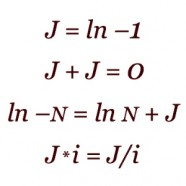# James ImaginaryStudy of the James algebra yields a new type of imaginary number J, the logarithm of negative one. This imaginary is simpler than the conventional imaginary value i, the square root of negative one, in that J has a period of two rather than four. The imaginary i is constructed from two out-of-phase instances of J. J is the additive imaginary, while i is the multiplicative imaginary. The Image shows some primary characteristics of J. Two copies of J add together to yield zero, even though J itself is not equal to zero. J provides a definition of the logarithm of negative numbers. Similar to i which defines a forbidden square root, J defines a forbidden logarithm. The significant results involving J, together with many computational applications for J, are contained in the forthcoming book, Iconic Arithmetic Volume III. The origin of the content presented on this page and on the James Algebra page is discussed in depth in Jeffrey James’ 1993 masters thesis from the University of Washington. The thesis, A Calculus of Number Based on Spatial Forms, is available online on the ancient Laws of Form website.

Note, October 2020: The James algebra content is being explored and greatly expanded in a series of three volumes of Iconic Arithmetic described on this site:

Iconic Arithmetic Volume I

Iconic Arithmetic Volume II

Iconic Arithmetic Volume III which is due in early 2021.